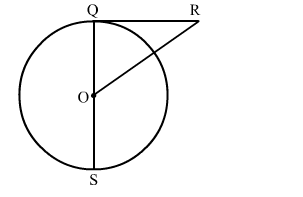# In the given figure, RQ is a tangent to the circle with centre O.`
Question:

In the given figure, RQ is a tangent to the circle with centre O. If SQ = 6 cm and QR = 4 cm, then OR is equal to

(a) 2.5 cm
(b) 3 cm
(c) 5 cm
(d) 8 cmSolution:

We know that the radius and tangent are perperpendular at their point of contact

$\mathrm{OQ}=\frac{1}{2} \mathrm{QS}=3 \mathrm{~cm}$        [∵Radius is half of diameter]

Now, in right triangle OQR
By using Pythagoras theorem, we have
OR2 = RQ2 + OQ2
= 42 + 32
= 16 + 9
= 25
∴OR2 = 25
⇒OR = 5 cm
Hence, the correct answer is option (c).